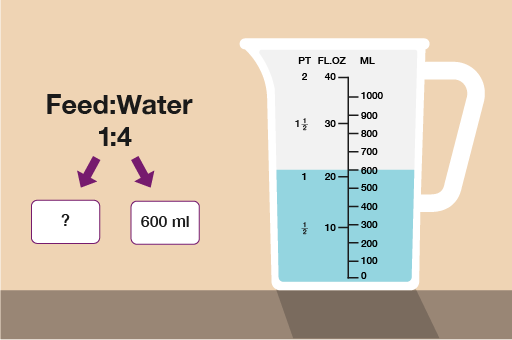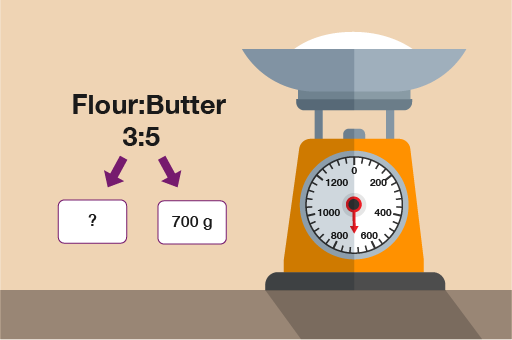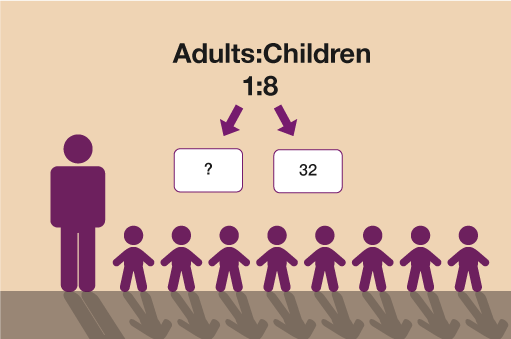Everyday maths for Health and Social Care and Education Support 2

Start this free course now. Just create an account and sign in. Enrol and complete the course for a free statement of participation or digital badge if available.

Free course

# 7.2 Solving ratio problems where the total of one part of the ratio is given

Take a look at the worked example below:

You are growing tomatoes. The instructions on the tomato feed say:

• Use 1 part feed to 4 parts water

If you use 600 ml of water, how much tomato feed should you use?

These questions make much more sense if you look at them visually:Figure 10 Solving ratio problems to grow tomatoes

You can now see clearly that 600 ml of water is worth 4 parts of the ratio. To find one part of the ratio you need to do:

• 600 ml ÷ 4 = 150 ml

Since the feed is only 1 part, feed must be 150 ml. If feed was more than one part you would multiply 150 ml by the number of parts.

## Activity 14: Ratio problems with one part given

Practise your skills by tackling the ratio problems below:

1. A recipe requires flour and butter to be used in the ratio 3:5. The amount of butter used is 700 g. How much flour will be needed?

2. When looking after children aged between 7 and 10, the ratio of adults to children must be 1:8.

• a.For a group of 32 children, how many adults must there be?

• b.If there was one more child in the group, how would this affect the number of adults required?

1. Flour:ButterFigure 11 Using ratios in recipes

To find one part you do 700 g ÷ 5 = 140 g

To find the amount of flour needed you then do 140 g × 3 = 420 g flour.

2. (a)Figure 12 Working out ratios of adults to children

To find one part you do 32 ÷ 8 = 4.

(b) If there were 33 children, one part would be 33 ÷ 8 = 4.125.

Since you cannot have 4.125 adults, you need to round up to 5 adults so you would need one more adult for 33 children.

FSM_SSH_2